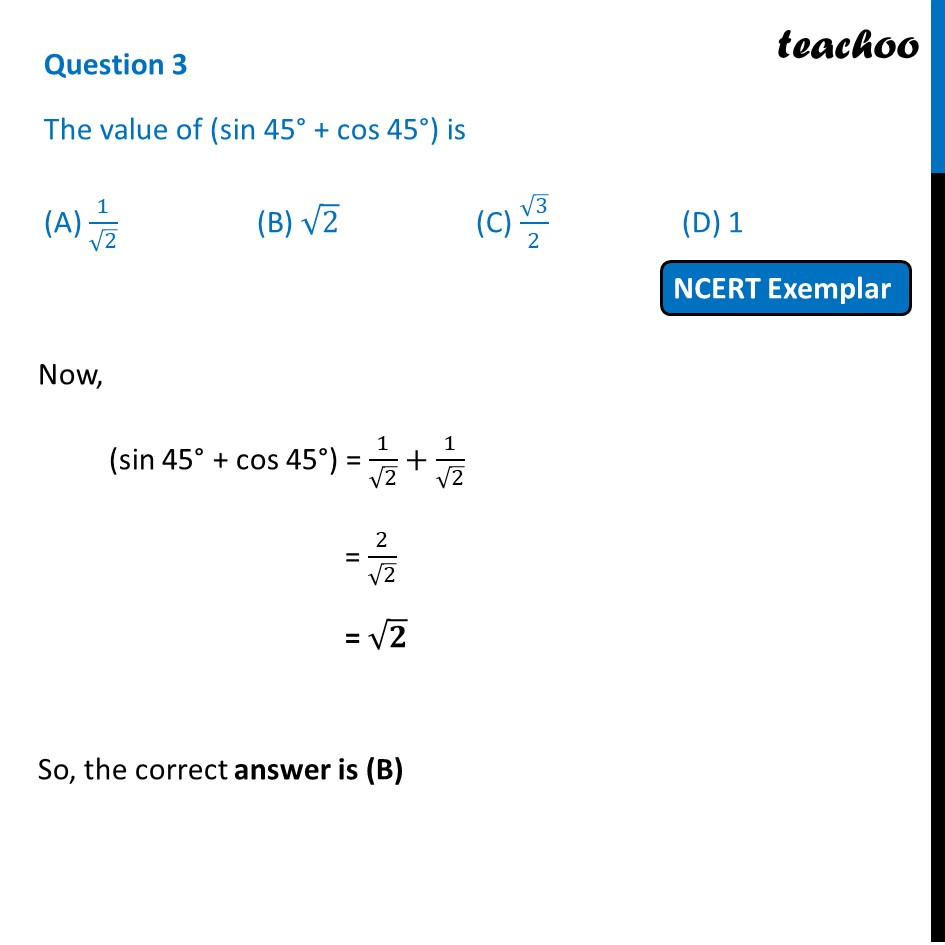## (A) 1/√2  (B) √2     (C) √3/2  (D) 11. Chapter 8 Class 10 Introduction to Trignometry (Term 1)
2. Serial order wise
3. NCERT Exemplar - MCQ

Transcript

Question 3 The value of (sin 45° + cos 45°) is (A) 1/√2 (B) √2 (C) √3/2 (D) 1 Now, (sin 45° + cos 45°) = 1/√2+1/√2 = 2/√2 = √𝟐 So, the correct answer is (B)